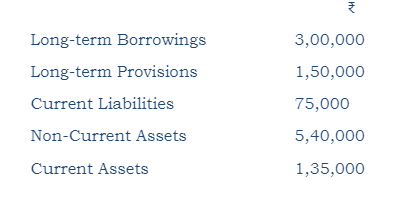(i) X Ltd. has a current ratio of 3 : 1 and quick ratio of 2 : 1. The excess of current assets over quick assets are 24,000. Calculate current assets and current liabilities.

(ii) From the following information, compute ‘Total Assets to Debt Ratio’:Marks-3, CBSE:2019-20/Compartment/Q-30*

error: Content is protected !!